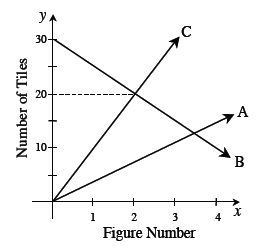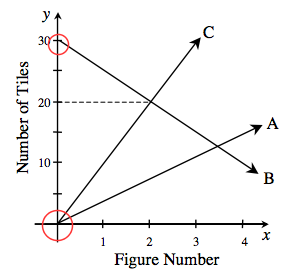### Home > AC > Chapter 4 > Lesson 4.1.6 > Problem4-55

4-55.

Examine the graph below showing three tile patterns.

1. What do you know about Figure 0 for each of the three patterns?

2. Which pattern changes most quickly? How quickly does it change? Show how you know.

3. Which figure number has the same number of tiles in patterns B and C? Explain how you know.

4. Write a rule for pattern B.What is the starting point for each pattern?

Figure 0 has
Pattern A: $0$ tiles
Pattern B: $30$ tiles
Pattern C: $0$ tilesFind the growth of each pattern.
For the pattern that changes the most quickly,
the growth will be the greatest.

Pattern C grows the most quickly at $10$ tiles per figure.
Its line is the steepest.

Where two lines on the graph intersect, the number of tiles and the figure number are the same for those two patterns.

Figure 2 (See the graph).

Use the starting point for pattern B you found in part (a) and the growth you found for pattern B in part (b).